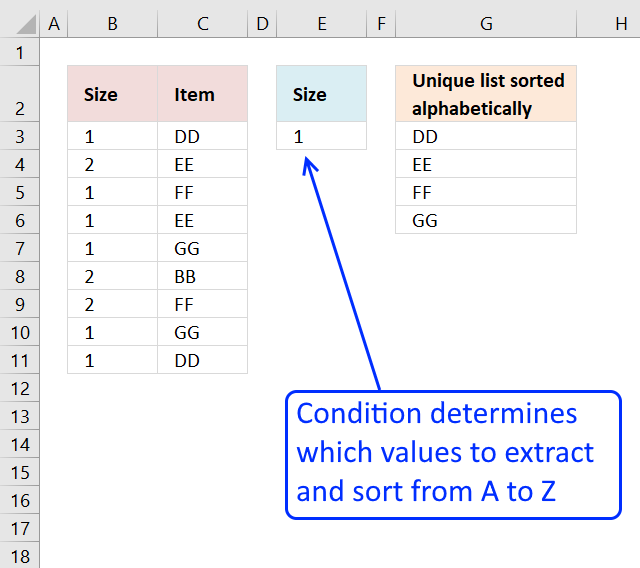Author: Oscar Cronquist Article last updated on December 17, 2020Update 17 December 2020, the new FILTER, UNIQUE, and SORT functions are now available for Excel 365 users.

=SORT(UNIQUE(FILTER(C3:C11, E3=B3:B11)))

This is entered as a regular formula, however, it returns an array of values and extends automatically to cells below and to the right. Microsoft calls this a dynamic array and spilled array.

The array formula below is for earlier Excel versions, it filters values in column C based on the value in cell E3, the output is a sorted unique distinct list in cell E6 and below.

Array formula in cell E6:

=INDEX(\$C\$3:\$C\$11, MATCH(SMALL(IF((COUNTIF(\$G\$2:G2, \$C\$3:\$C\$11)=0)*(\$B\$3:\$B\$11=\$E\$3), COUNTIF(\$C\$3:\$C\$11, "<"&\$C\$3:\$C\$11), ""), 1), COUNTIF(\$C\$3:\$C\$11, "<"&\$C\$3:\$C\$11), 0))

Recommended post

#### How to create an array formula

1. Double click on cell E6
2. Copy (Ctrl + c) and paste (Ctrl + v) array formula to cell
3. Press and hold Ctrl + Shift simultaneously
4. Press Enter once.
5. Release all keys.

There are now a beginning and ending curly bracket in the formula bar, like this: {=formula}
Don't enter these characters yourself.

#### Explaining array formula in cell E6

Read my explanation here: Create a unique distinct alphabetically sorted list, extracted from a column

Recommended reading:

### Download excel *.xlsx file

Create a unique distinct alphabetically sorted list with criteria.xlsx